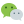# 概念

``````1 1 2 3 5 8 13 21 34...
``````

# 斐波那契数

``````function getFebNum(n) {
if (n == 1 || n == 2) {
return 1;
} else {
return getFebNum(n - 1) + getFebNum(n - 2);
}
}
``````

``````function _getFebNum(n) {
if (n < 1) return 0;
let one = 1, // 初始为第 -2 项
two = 0, // 初始为第 -1 项
three = 0; // 初始为第 1 项
for (let i = 1; i <= n; i++) {
three = one + two;
one = two;
two = three;
}
return three;
}
``````

-2 -1 1 2 3 4 5
1 0 1 1 2 3 5

# 斐波那契数列

``````function getFebArr(n) {
let arr = [];
for (let i = 1; i <= n; i++) {
arr.push(getFebNum(i));
}
return arr;
}
``````

``````function _getFebArr(n) {
let arr = [];
if (n < 1) return arr;
let one = 1,
two = 0,
three = 0;
for (let i = 1; i <= n; i++) {
three = one + two;
arr.push(three);
one = two;
two = three;
}
return arr;
}
``````

### 评论：微信公众号：程序骑士